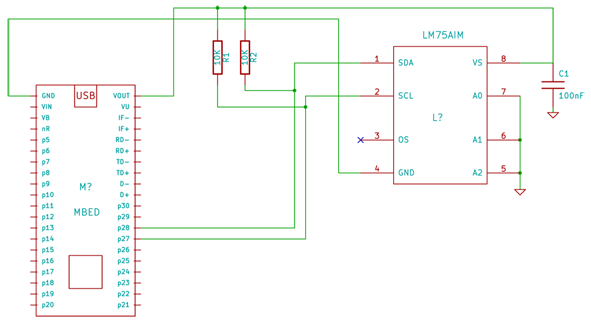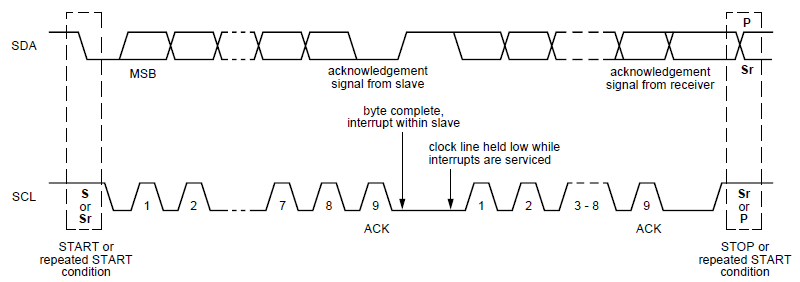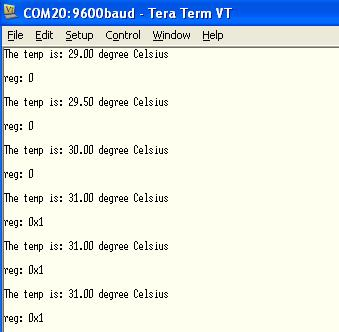LM75AIM Temperature Sensor

The LM75AIM is an I2C digital temperature sensor in a small SOP8 package.

Datasheet

Features:

Operating Voltage Range: +Vs = 2.7V to 5.5V
Temperature Measurement Range: -25°C to +100°C (accuracy of ±2°C)
Interface: I2C (up to 400KHz)
Resolution: 9bit

Connection:

LM75A pinMbed pin
1 - SDAP28
2 - SCLP27
3 - OSNC
4 - GNDGND
5 - A2GND
6 - A1GND
7 - A0GND
8 - VsVout

Schematic:The I2C bus requires a 10Kohm pull-up resistor.
R1 and R2 = 10Kohm
C1 = 100nF
The pins A0 to A2 are used for set a I2C Address of this chip.
I set the pins A0, A1, A2 to "zero" so my I2C Address Slave is: 1001000 (0x48)

I2C:

The I2C bus is based on two lines: serial data (SDA) and serial clock (SCL).
Every byte put on the SDA line must be 8bit long.

After the START condition (S), a Slave Address is sent and it consists of 7bit followed by an eighth bit which is a data direction bit (R/W).
If the data direction bit is "zero" this indicate a transmission (W), but if it is "one" this indicate a request for data (R).
Then the data are send.
A data transfer is always terminated by a STOP condition (P) generated by the Master.Test Code:

The complete I2C Address Slave is: 10010000 (0x90)

For read the temperature from the sensor I need to select the Temperature Pointer Register (0x00).

Then I can read the temperature data that is represented by 9bit (if the LSB bit is "1" it is equal to 0.5°C).

Import programLM75A_Test_Code

Test code for LM75AIM

Library:

Import libraryLM75A

Library for the LM75A Temperature Sensor

Output:

The value of the register 0x01 changes after three cycles.Update: Add LM75A Library and new Test Code